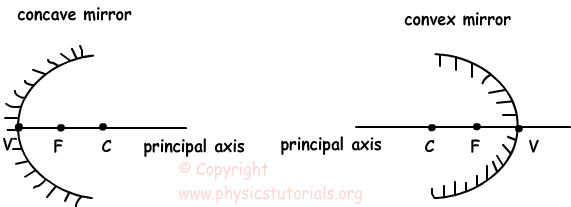## Curved Mirrors

Curved Mirrors

We call these types of mirrors also spherical mirrors because they are pieces of a sphere. If the reflecting surface of the mirror is outside of the sphere then we call it convex mirror and if the reflecting surface of it is inside the sphere then we call it concave mirror. There are some fundamental terms we should learn before we pass to the ray diagrams and image formation in curved mirrors such as principal axis, focal point, center of curvature, radius of curvature and vertex.Center of Curvature: As you can understand from the name it is the center of the sphere which the mirror is taken from. It is denoted by C in the diagrams.

Principal Axis: Line coming from the center of the sphere to the mirror is called as principal axis.

Vertex: It is the intersection point of the mirror and principal axis. We show it with the letter V in ray diagrams.

Focal Point: For concave mirrors and thin lenses rays coming parallel to the principal axis reflects from the optical device and pass from this point. For convex mirrors and thick lenses rays coming from this point or appear to coming from this point reflect parallel to the principal axis from the optical device. Another explanation for this term is that, it is the point where the image of the object at infinity is formed. It is denoted with the letter F or sometimes f in ray diagrams.

Radius of Curvature: It is the distance between center of the sphere and vertex. We show it with R in ray diagrams.

Optics Exams and Solutions

Author: